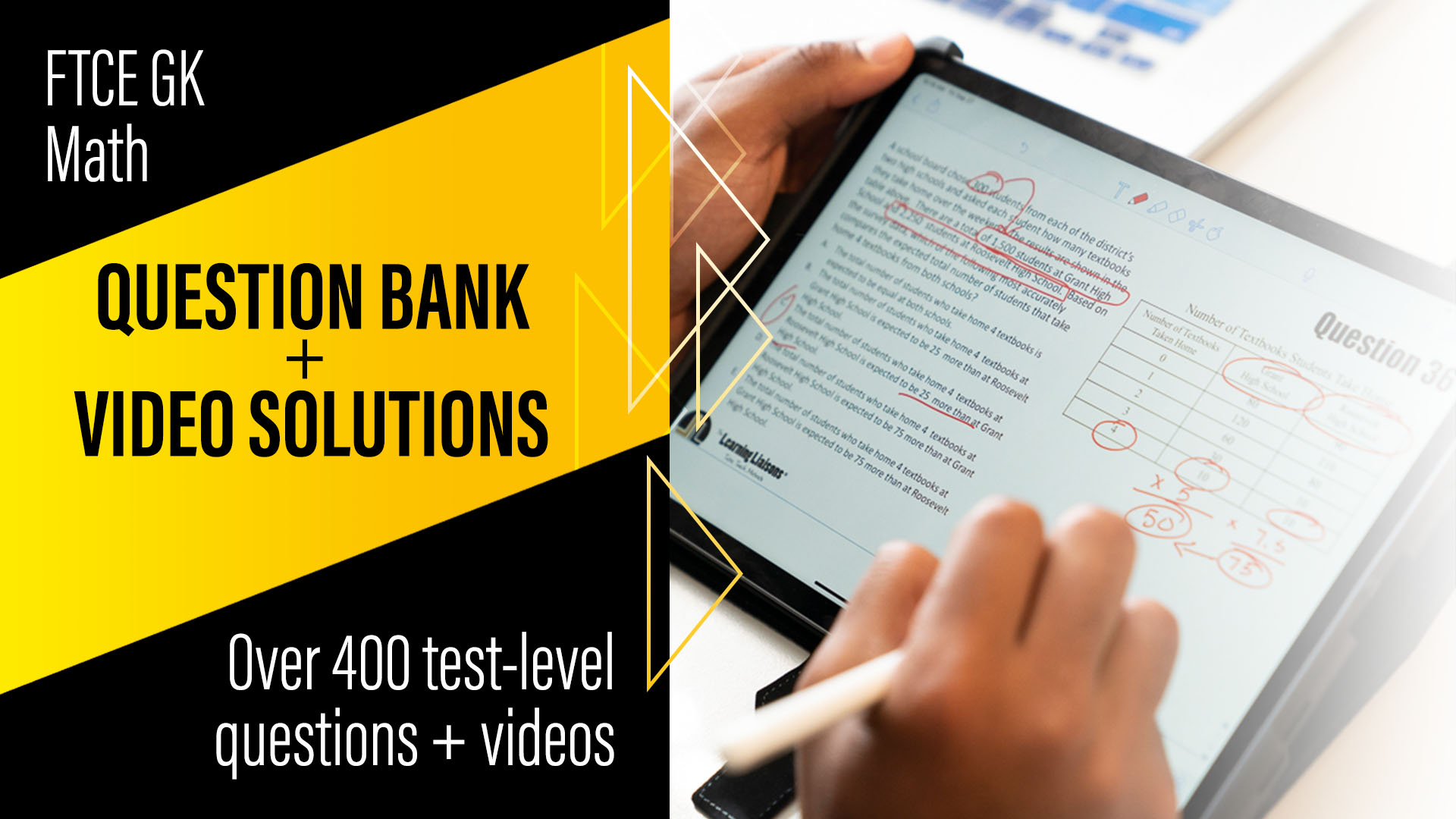### The Learning Liaisons: GK Math Practice Questions

#### \$19.99 a month or \$50 a year after 3-day free trial

It shouldn’t be hard to find great practice material for the FTCE General Knowledge Mathematics Subtest. We understand the pain of learning math, especially if you’ve struggled with math for a long time.

After helping 6000 teachers pass the FTCE GK Math test, we know we can help you pass too. Use this FTCE GK Math video channel to access over 300 test-aligned practice questions and video solutions. With this question bank, you can clear up the confusion and gain the knowledge, skill, and attitude needed to pass on your next attempt!

Use these practice problems in 3 easy steps:
1. Pick a competency and skill
2. Open and pause the video so you can try the problem out
3. Press “play” when you’re ready and learn how it’s done!

Avoid the confusion of practicing with the wrong test preparation material and pass the FTCE GK Math test by signing up today.

FTCE GK Math Competencies and Skills Blueprint:

Competency 1—Knowledge of number sense, concepts, and operations
1. Compare real numbers and identify their location on a number line.
2. Solve real-world problems involving the four operations with rational numbers.
3. Evaluate expressions involving order of operations.

Competency 2—Knowledge of geometry and measurement
1. Identify and classify simple two- and three-dimensional figures according to their mathematical properties.
2. Solve problems involving ratio and proportion (e.g., scaled drawings, models, real-world scenarios).
3. Determine an appropriate measurement unit and form (e.g., scientific notation) for real-world problems involving length, area, volume, or mass.
4. Solve real-world measurement problems including fundamental units (e.g., length, mass, time), derived units (e.g., miles per hour, dollars per gallon), and unit conversions.

Competency 3—Knowledge of algebraic thinking and the coordinate plane
1. Determine whether two algebraic expressions are equivalent by applying properties of operations or equality.
2. Identify an algebraic expression, equation, or inequality that models a real-world situation.
3. Solve equations and inequalities (e.g., linear, quadratic) graphically or algebraically.
4. Determine and solve equations or inequalities, graphically or algebraically, in real-world problems.
5. Graph and interpret a linear equation in real-world problems (e.g., use data to plot points, explain slope and y-intercept, determine additional solutions).
6. Identify relations that satisfy the definition of a function.
7. Compare the slopes of two linear functions represented algebraically and graphically.

Competency 4—Knowledge of probability, statistics, and data interpretation
1. Analyze data presented in various forms (e.g., histograms, bar graphs, circle graphs, pictographs, line plots, tables) to solve problems.
2. Analyze and evaluate how the presentation of data can lead to different or inappropriate interpretations in the context of a real-world situation.
3. Calculate range, mean, median, and mode of data sets.
4. Interpret the meaning of measures of central tendency (i.e., mean, median, mode) and dispersion (i.e., range, standard deviation) in the context of a real-world situation.
5. Analyze and evaluate how the selection of statistics (e.g., mean, median, mode) can lead to different or inappropriate interpretations in the context of a real-world situation.
6. Solve and interpret real-world problems involving probability using counting procedures, tables, and tree diagrams.
7. Infer and analyze conclusions from sample surveys, experiments, and observational studies.